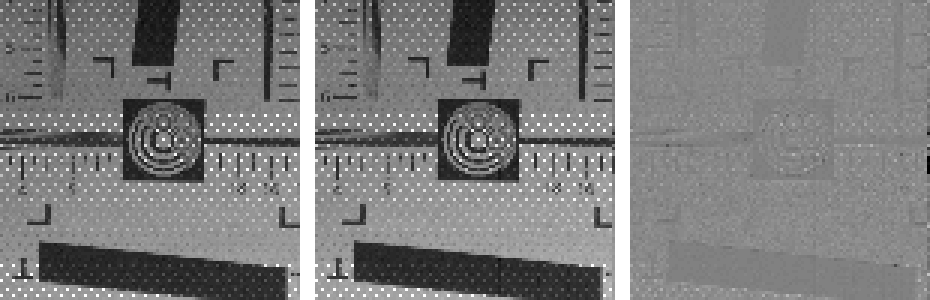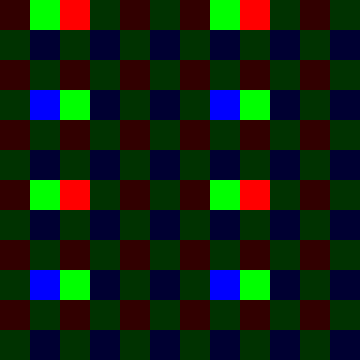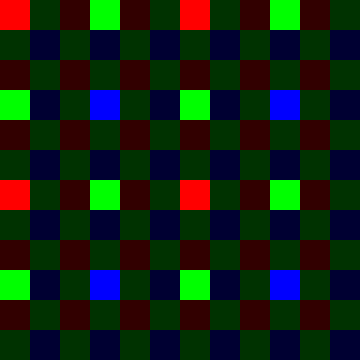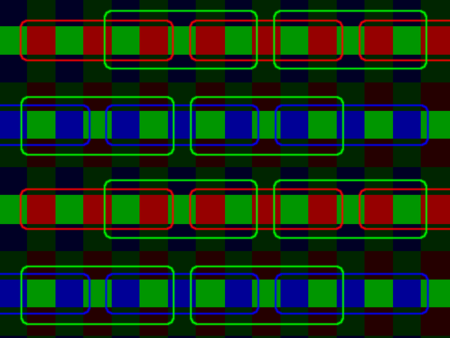### Mysterious "extreme moiré" 3x3 readout on the EOS M¶

Bug reported here.

I would say even worse... the moiree is so extreme, that you can see it in liveview already.

Best guess: this must be 3x3 readout with line and column skipping!

Test images here. One is 3x3 "extreme moiré" readout, the other is 1:1 readout from x5 zoom. No camera motion between the two images.

In :
%%shell
wget -c -q https://www.dropbox.com/s/70ig9z535kixmk0/binningtest3.zip?dl=1 -O binningtest3.zip
unzip binningtest3.zip

Archive:  binningtest3.zip
inflating: 98180001.DNG
inflating: 98180002.DNG

In :
pkg load image



Some helper functions to display the images:

In :
# raise the shadows
function y = gamma(x)
y = sqrt(min(max(x - 2048, 0), 5000));
end

# show image
function show(x)
imshow(gamma(x), [])
end

# show image, with zoom (integer factor, nearest neighbour interpolation)
function show_z(x, zoom)
imshow(imresize(gamma(x), zoom, 'nearest'), [])
end

# show image difference, with zoom
function show_dz(a, b, zoom)
imshow(imresize(a - b, 4, 'nearest'), [-100 100])
end

# blank space between 2 images
function y = blank(x)
y = zeros(size(x,1), round(size(x,1)/20)) + inf;
end

# show 2 images side by side
function cmp(a, b, zoom, dif)
if nargin > 3
show_z([a, blank(a), b, blank(a), b - a + 2048 + (sqrt(5000)/2)^2], zoom)
#    imshow(imresize([gamma([a, blank(a), b]), blank(a), b - a], zoom, "nearest"), [])
else
show_z([a, blank(a), b], zoom)
end
end


Let's extract a crop from each image (manual tweaking):

In :
ac = a(401:600,751:950);
bc = b(283:882, 809:1408);

cmp(ac, imresize(bc,1/3), 2)Hypothesis: from each 3x3 pixel block of the same Bayer color, only one pixel is captured. The other 8 are discarded.

In :
for i = [1 3],
for j = [1 3],
figure, cmp(ac, bc(i:3:end, j:3:end), 2)
end
endNope, the moiré is much worse than that!

Let's look at individual Bayer channels.

In :
# trial and error - good match (red channel)
cmp(ac(1:2:end, 1:2:end), bc(1:6:end,3:6:end), 3, 1)In :
# trial and error - another good match (green1 channel)
cmp(ac(1:2:end, 2:2:end), circshift(bc(1:6:end,2:6:end), [0,-1]), 3, 1)In :
# some bad match (green1 channel)
cmp(ac(1:2:end, 2:2:end), bc(1:6:end,6:6:end), 3, 1)That seems to give a good match. Maybe each Bayer channel has its own phase (i.e. position of the active pixel in the 3x3 grid) ?

Let's find out. When all else fails, brute force prevails.

In :
# brute-force scanning to find the binning mode
# hypothesis: only one pixel from a 3x3 group is going to be used
# we want to find which pixel (of these 3x3), for each Bayer channel

# create a Bayer grid for showing the result
binmode(:,:,1) = zeros(size(bc));
binmode(:,:,2) = zeros(size(bc));
binmode(:,:,3) = zeros(size(bc));
binmode = uint8(binmode * 255);
colors = [1 2; 2 3];

# set all channels to "unused" (washed out)
for di = 1:2
for dj = 1:2
binmode(di:2:end, dj:2:end, colors(di,dj)) = 50;
end
end

# for each Bayer channel
for di = 1:2
for dj = 1:2

# find the best match
# we may also want to shift the image a bit to keep alignment
# search space is small, so we can just brute-force things
E = [];
for dr = 1:6
for dc = 1:6
for ci = -2:2
for cj = -2:2
E(dr,dc,ci+3,cj+3) = norm((ac(di:2:end, dj:2:end) - circshift(bc(dr:6:end, dc:6:end), [ci, cj]))(11:end-10, 11:end-10)(:));
end
end
end
end

# show the best match
[m,ind] = min(E(:));
[dr,dc,ci,cj]=ind2sub(size(E), ind); ci -= 3; cj -= 3;
printf("channel (%d,%d) phase (%d,%d) offset (%d,%d)\n", di, dj, dr, dc, ci, cj)
figure, cmp(ac(di:2:end, dj:2:end), circshift(bc(dr:6:end, dc:6:end), [ci, cj]), 3, 1)

# mark it on the Bayer map
binmode(dr:6:end, dc:6:end, colors(di,dj)) = 255;
end
end

channel (1,1) phase (1,3) offset (0,0)
channel (1,2) phase (1,2) offset (0,-1)
channel (2,1) phase (4,3) offset (0,0)
channel (2,2) phase (4,2) offset (0,-1)So far, so good; let's see the results.

In :
# for some reason, imshow will display a dark image, figure out why
# workaround: imagemagick + markdown
imwrite(binmode(1:12, 1:12, :), 'binmode.png');
system("mogrify -filter Point -resize 3000% binmode.png");Ta-da!

It is, indeed, 3x3 readout with line/column skipping. Only one pixel from each 3x3 group (of pixels with the same Bayer color) is captured. The other 8 are skipped.

However, the distance between the pixels actually read out is not uniform on the X direction. Rather, every two columns are adjacent.

Ideally, a 3x3 readout with line/column skipping would have been like this:With the "extreme moiré" readout, the same number of pixels is being captured. However, since we may have 4 columns skipped between two captured pixels, the Nyquist frequency is much lower.

Overall, aliasing in this "extreme moiré" mode is worse than in 720p, where a 5x3 readout (line skipping / column binning) is used. There, the camera reads out 3 pixels out of each 5x3 block of pixels having the same Bayer color.

With regular 1080p 3x3, most Canons use the following pixel binning method (source):Does that mean the pixel binning phase, at least horizontally, can be adjusted by software?

That could be pretty interesting.